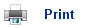# Shapes of Molecules

• Dot and cross diagrams can be used to describe the layout of molecules. However it has its limitations as it only shows the two dimensional layout of the atoms, and not the three dimensional shape.
• All electrons have a negative charge; like charges repel, so the electrons are arranged so that they are as far away as possible from each other. This is what determines the shape of molecules.
• It is not only the shared electrons that affect the shape of the molecule, but the lone pairs also affect the shape, in fact they have a greater effect as their negative charge is stronger than that of the shared electrons.
• Balloons can be used to represent the layout of different molecules: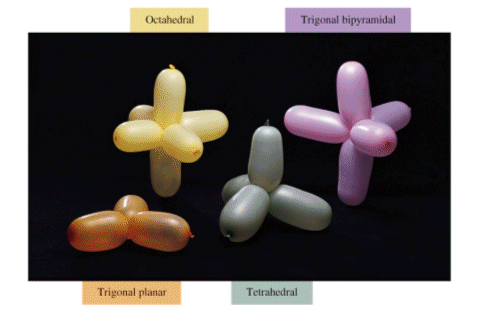• The various shapes of the molecules are as follows:
• Triangular Planar.
• Tetrahedral.
• V-shaped.
• Linear.
• Pyramidal.

## Triangular Planar

• Boron tri-fluoride (BH3) has a dot and cross diagram as below: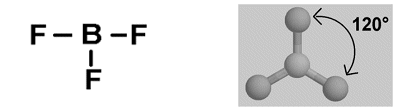• The bonding electron pairs around the central Boron atom have the same repulsion, and so an equilateral triangle is formed around the central Boron molecule. This means that the angle between the fluorine molecules is 120o.

## Tetrahedral

• Methane (CH4) has a dot and cross diagram as below: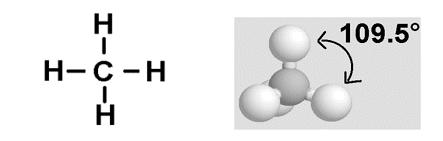• The four bonding electron pairs around the central carbon atom in this molecule have the same negative repulsion, and so they are formed so that they are as far apart as possible. The angle found between the molecules in 109.5o.

## V-shaped

• Water (H2O) has a dot and cross diagram as below:• In this molecule around the central oxygen atom, the shared pair of electrons repel each other, but the two lone electrons also repel the two shared pairs and so a v-shape is formed, with an angle of 104.4o in between the atoms.

## Linear

• Beryllium chloride (BeCl2) has a dot and cross diagram as below: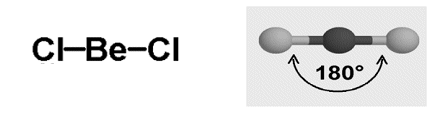• As there are only two bonding pairs of electrons, they are found directly opposite each other.

## Pyramidal

• Ammonia (NH3) has a dot and cross diagram as below: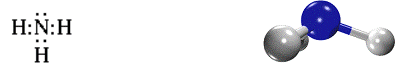• The lone pair of electrons around the central Nitrogen atom repel the three bonding electron pairs forming a pyramid shape, with an angle of 107o between each atom.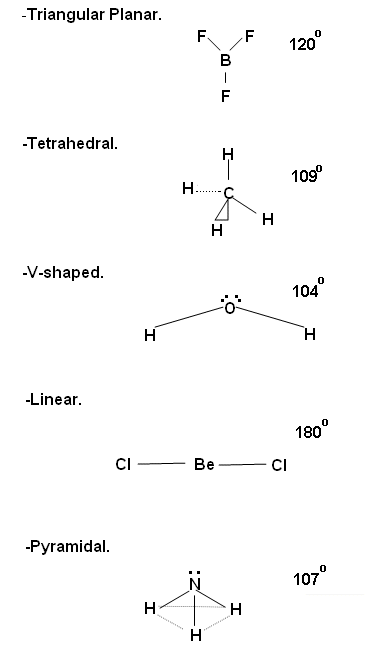## Useful books for revision:

Revise AS Chemistry for Salters (Written by experienced examiners and teachers of Salter's chemistry)Revise AS Chemistry for Salters (OCR) (Salters Advanced Chemistry)Home